SAT  >  Test: Lines & Angles - 1

# Test: Lines & Angles - 1

Test Description

## 10 Questions MCQ Test Quantitative for GMAT | Test: Lines & Angles - 1

Test: Lines & Angles - 1 for SAT 2023 is part of Quantitative for GMAT preparation. The Test: Lines & Angles - 1 questions and answers have been prepared according to the SAT exam syllabus.The Test: Lines & Angles - 1 MCQs are made for SAT 2023 Exam. Find important definitions, questions, notes, meanings, examples, exercises, MCQs and online tests for Test: Lines & Angles - 1 below.
Solutions of Test: Lines & Angles - 1 questions in English are available as part of our Quantitative for GMAT for SAT & Test: Lines & Angles - 1 solutions in Hindi for Quantitative for GMAT course. Download more important topics, notes, lectures and mock test series for SAT Exam by signing up for free. Attempt Test: Lines & Angles - 1 | 10 questions in 20 minutes | Mock test for SAT preparation | Free important questions MCQ to study Quantitative for GMAT for SAT Exam | Download free PDF with solutions
 1 Crore+ students have signed up on EduRev. Have you?
Test: Lines & Angles - 1 - Question 1

### In the figure given below, ABC is a triangle. BC is parallel to AE. If BC = AC, then what is the value of ∠CAE?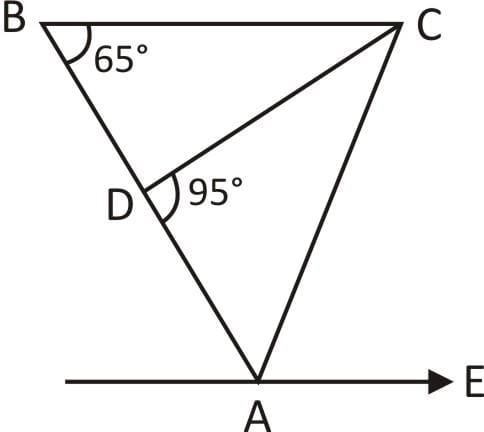Detailed Solution for Test: Lines & Angles - 1 - Question 1

Given that, BC || AE
∠CBA + ∠EAB = 180°
⇒ ∠EAB = 180° – 65° = 115°
∵   BC = AC
Hence, ΔABC is an isosceles triangle.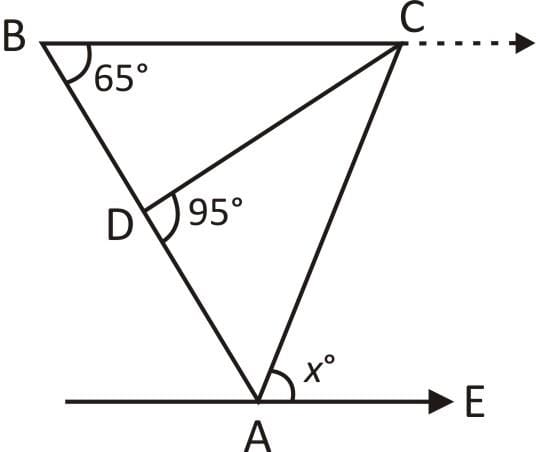⇒ ∠CBA = ∠CAB = 65°
Now,  ∠EAB = ∠EAC + ∠CAB
⇒ 115° = x + 65° ⇒ x = 50°.
Hence, option D is correct.

Test: Lines & Angles - 1 - Question 2

### In the figure given below, AB is parallel to CD. ∠ABC = 65°, ∠CDE = 15° and AB = AE. What is the value of ∠AEF?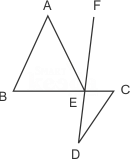Detailed Solution for Test: Lines & Angles - 1 - Question 2

Given that,

∠ABC = 65° and ∠CDE = 15°

Here, ∠ABC + ∠TCB = 180°

(∵   AB || CD)

∠TCB = 180° – ∠ABC

∴ ∠TCB = 180° – 65° = 115°

∵ ∠TCB + ∠DCB = 180°

(Linear pair)

∴ ∠DCB = 65°

Now, in ΔCDE

∠CED = 180° – (∠ECD + ∠EDC)

(∵ ∠ECD = ∠BCD)

= 180° – (– 65° + 15° ) = 100°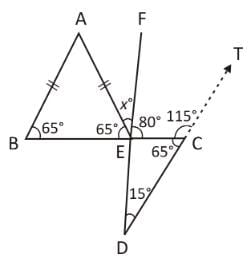∵ ∠DEC + ∠FEC = 180°

⇒ ∠FEC = 180° – 100° = 80°

Given that, AB = AE.

i.e. ΔABE an isosceles triangle.

∴ ∠ABE = ∠AEB = 65°

∵ ∠AEB + ∠AEF + ∠FEC = 180°

(straight line)

⇒ 65° + x° + 80° = 180°

∴ x° = 180° – 145° = 35°.

Hence, option B is correct.

Test: Lines & Angles - 1 - Question 3

### The angles x°, a°, c° and (π – b)° are indicated in the figure given below. Which one of the following is correct?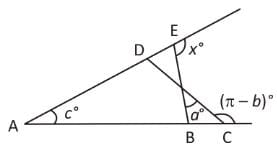Detailed Solution for Test: Lines & Angles - 1 - Question 3

∠PCT + ∠PCB = π
(Linear pair)

∠PCB = π – (π – b°) = b° ..... (i)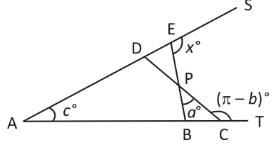In ΔBPC,

∠PCB + ∠BPC + ∠PBC = π

∠PBC = π – ∠PCB – ∠BPC  =  π – b° – a° ..... (ii)

∵ ∠ABE + ∠EBC = π
(∵ ∠PBC = ∠EBC)      (linear pair)
∠ABE = π – ∠PBC = π – (π – b° – a°)  =  a° + b° ...(iii)

Now, in ΔABE

Sum of two interior angles = Exterior angle

∠EAB + ∠ABE = ∠BES ⇒ c° + b° + a° = x°

∴   x° = a° + b° + c°.

Hence, option C is correct.

Test: Lines & Angles - 1 - Question 4

Consider the following statements

I. The locus of points which are equidistant from two parallel lines is a line parallel to both of them and drawn mid way between them.
II. The perpendicular distance of any point on this locus line from two original parallel lines are equal. Further, no point outside this locus line has this property.

Which of the above statements is/are correct?

Detailed Solution for Test: Lines & Angles - 1 - Question 4

Statements I and II are both true, because the locus of points which are equidistant from two parallel lines is a line parallel to both of them and draw mid way between them.

Also, it is true that the perpendicular distances of any point on this locus line from two original parallel lines are equal. Further, no point outside this locus line has this property.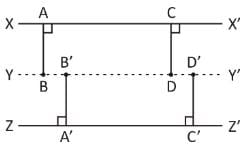Hence, option C is correct.

Test: Lines & Angles - 1 - Question 5

A wheel makes 12 revolutions per min. The angle in radian described by a spoke of the wheel in 1 s is:

Detailed Solution for Test: Lines & Angles - 1 - Question 5

= 12 × Its circumference
= 12 × 2πr
∴  In 1 s distance travelled by the wheel =
(12 × 2πr) / 60 = (2 / 5) πr
∴ Angle = (Arc / Radius)
= (2 / 5πr) / r = 2π / 5
Which is the required angle.
Hence, option B is correct.

Test: Lines & Angles - 1 - Question 6

If one arm of an angle is respectively parallel to the arm of another angle formed with a common line between them, then the two angles are

Detailed Solution for Test: Lines & Angles - 1 - Question 6

If one arm of an angle is respectively parallel to the arm of another angle formed with a common line between them, then the two angles are not equal but supplementary.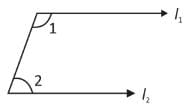If l1 || l2 ⇒ ∠1 + ∠2 = 180°
(Supplementary)

Hence, option B is correct.

Test: Lines & Angles - 1 - Question 7

In a Δ ABC, (1 / 2) ∠A + (1 / 2) ∠C + (1 / 2) ∠B = 80°, then what is the value of ∠C?

Detailed Solution for Test: Lines & Angles - 1 - Question 7

Given that,
(1 / 2) ∠A + (1 / 2) ∠C + (1 / 2) ∠B = 80°
⇒   3∠A + 2∠C + 3∠B = 480°
⇒   3(∠A + ∠B) + 2∠C = 480° ....(i)
Also, in ΔABC,
∠A + ∠B + ∠C = 180°
On multiplying both sides L.H.S. & R.H.S. by 3, we get
3(∠A + ∠B) + 3∠C = 540°  ..... (ii)
On subtracting Eq. (i) from Eq. (ii), we get
∠C = 60°.
Hence, option C is correct.

Test: Lines & Angles - 1 - Question 8

The complement angle of 80° is

Detailed Solution for Test: Lines & Angles - 1 - Question 8

Complementary angles: Complementary angles are angle pairs whose measures sum to one right angle (90°).

So, the required angle will be 10°
1º = (π / 180)
∴ 10º = {(π × 10) / 180} = (π / 18)
Hence, option C is correct.

Test: Lines & Angles - 1 - Question 9

In the given figure AB || CD, ∠ALC = 60° and EC is the bisector of ∠LCD. If EF || AB then the value of ∠CEF is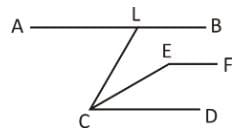Detailed Solution for Test: Lines & Angles - 1 - Question 9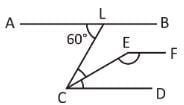∠ALC = ∠LCD = 60°
[∵  Alternate angles]
EC is the bisector of ∠LCD
∴ ∠ ECD = (1 / 2) × ∠ LCD
= (1 / 2) × 60º = 30º
∠CEF + ∠ECD = 180°
[∵  Pair of interior angles]
∠CEF + 30° = 180°
∠CEF = 180° – 30° = 150°
Hence, option C is correct.

Test: Lines & Angles - 1 - Question 10

In the given figure lines AP and OQ intersect at G. If ∠AGO + ∠PGF = 70° and ∠PGQ = 40°. Find the angle value of ∠PGF.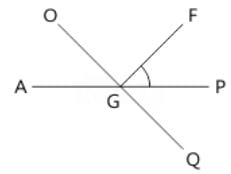Detailed Solution for Test: Lines & Angles - 1 - Question 10

As AP is a straight line and rays GO and GF stands on it.
∴ ∠AGO + ∠OGF + ∠PGF = 180°

⇒ (∠AGO + ∠PGF) + ∠OGF = 180°

⇒ 70° + ∠OGF = 180°

⇒ ∠OGF = 180° – 70°

⇒ ∠OGF = 110°

As, OQ is a straight line, rays GF and GP stands on it.

∠OGF + ∠PGF + ∠PGQ = 180°

Putting the value of ∠OGF & ∠PGQ

110° + ∠PGF + 40° = 180°

∠PGF = 180° – 150° = 30°
Hence, option C is correct.

## Quantitative for GMAT

88 videos|99 docs|165 tests
 Use Code STAYHOME200 and get INR 200 additional OFF Use Coupon Code
Information about Test: Lines & Angles - 1 Page
In this test you can find the Exam questions for Test: Lines & Angles - 1 solved & explained in the simplest way possible. Besides giving Questions and answers for Test: Lines & Angles - 1, EduRev gives you an ample number of Online tests for practice

## Quantitative for GMAT

88 videos|99 docs|165 tests